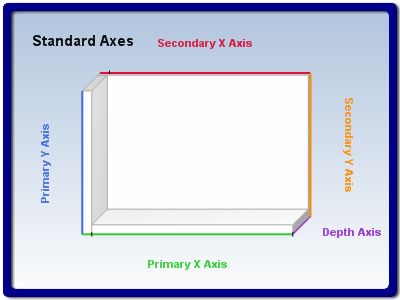﻿ Axes Types
Nevron .NET Vision Documentation
Axes Types

Chart and Gauge axes are represented by NAxis objects that reside in an axis collection. Cartesian charts have five built-in axes that are always present in the axis collection. Radar and Polar charts have only one axis object, while Pie, Funnel and Venn charts do not have any axes. Gauges have one axis by default.
Cartesian Chart Axes

The following figure displays the default position and orientation of the standard Cartesian axes:figure 1.

The Y axes are named StandardAxis.PrimaryY and StandardAxis.SecondaryY and are used to scale the series in the Y dimension. By default all series are scaled on the PrimaryY axis, while the SecondaryY axis is not visible.

The X axes are named StandardAxis.PrimaryX and StandardAxis.SecondaryX and are used to scale the series in the X dimension. By default all series are scaled on the PrimaryX axis, while the SecondaryX axis is not visible.

There is only one Z axis called StandardAxis.Depth axis. It is used in 3D charts to scale the series along the Z (depth) dimension. All chart series are scaled on the depth axis.

You can obtain a reference to one of the standard axes with the help of the Axis method of the NChart class.

The following code obtains a reference to the Secondary X axis object:

C#
Copy Code
```// obtain a reference to the Cartesian chart that is created by default
NCartesianChart chart = (NCartesianChart)chartControl.Charts;
// obtain a reference to the axis
NAxis axis = chart.Axis(StandardAxis.SecondaryX);
```
Visual Basic
Copy Code
```' obtain a reference to the Cartesian chart that is created by default
Dim chart As NCartesianChart = chartControl.Charts(0)
' obtain a reference to the axis
Dim axis As NAxis = chart.Axis(StandardAxis.SecondaryX)
```
Polar Chart Axes

Polar charts have only one axis, which is represented by an object of type NPolarAxis. The Polar axis implements the following specific features:

Control over the polar radial lines - the BeginAngle and AngleStep properties of the NPolarAxis class help you specify the angle on which the first radian line is displayed as well as the angle step for subsequent radian lines. The following code will display radian lines beginning from 30 degrees with step 33 degrees.

C#
Copy Code
```NPolarAxis polarAxis = (NPolarAxis)polarChart.Axis(StandardAxis.Polar);
polarAxis.BeginAngle = 30;
polarAxis.AngleStep = 33;
```
Visual Basic
Copy Code
```Dim polarAxis As NPolarAxis = polarChart.Axis(StandardAxis.Polar)
polarAxis.BeginAngle = 30
polarAxis.AngleStep = 33
```

Control over the polar radial texts - the Polar chart displays a label with the angle of each radial line at the line's end. You can control whether the displayed angle is in radians or degrees with the help of the AngleLabelsFormat property. By default it is set to <degree>. The following code will change the angle texts to radians.

C#
Copy Code
```polarAxis.AngleLabelsFormat = "<radian>";
```
Visual Basic
Copy Code
```polarAxis.AngleLabelsFormat = "<radian>"
```

The format of the displayed value is controlled by the AngleValueFormatter property. The following code will display the radian angle with 3 digits after the decimal point:

C#
Copy Code
```polarAxis.AngleValueFormatter = new NNumericValueFormatter("0.000");
```
Visual Basic
Copy Code
```polarAxis.AngleValueFormatter = New NNumericValueFormatter("0.000")
```

Control over the polar radian texts appearance and position - you can control the detachment of the polar texts from their original position with the help of the AngleLabelsDetachment property. The following code will increase the distance of the texts from the polar rim:

C#
Copy Code
```polarAxis.AngleLabelsDetachment = 6.0f;
```
Visual Basic
Copy Code
```polarAxis.AngleLabelsDetachment = 6.0F
```

The text appearance is controlled by the NTextStyle object accessible through the AngleLabelsTextStyle property.

C#
Copy Code
```polarAxis.AngleLabelsTextStyle.FontStyle = new NFontStyle("Arial", 10);
```
Visual Basic
Copy Code
```polarAxis.AngleLabelsTextStyle.FontStyle = New NFontStyle("Arial", 10)
```

Radar charts have only one axis, which is represented by an object of type NRadarAxis. The Radar axis implements the following specific features:

Control over the radar category texts - by default the radar axis displays numbers as category labels (1, 2, 3 etc.). You can easily override this behavior with the help of the AutoRadarLabels and RadarLabels properties of the radar axis. The following code will display custom labels for a radar chart with 5 categories:

C#
Copy Code
```NRadarAxis radarAxis = (NRadarAxis)radarChart.Axis(StandardAxis.Radar);
```
Visual Basic
Copy Code
```Dim radarAxis As NRadarAxis = radarChart.Axis(StandardAxis.Radar)
```

The appearance and position of the radar category texts is controlled through the RadarLabelsTextStyle and RadarLabelsDetachment properties. The following example changes the color of the category texts as well as increase their distance from the radar rim:

C#
Copy Code
```radarAxis.RadarLabelsTextStyle.FillStyle = new NColorFillStyle(Color.Red);
```
Visual Basic
Copy Code
```radarAxis.RadarLabelsTextStyle.FillStyle = New NColorFillStyle(Color.Red)